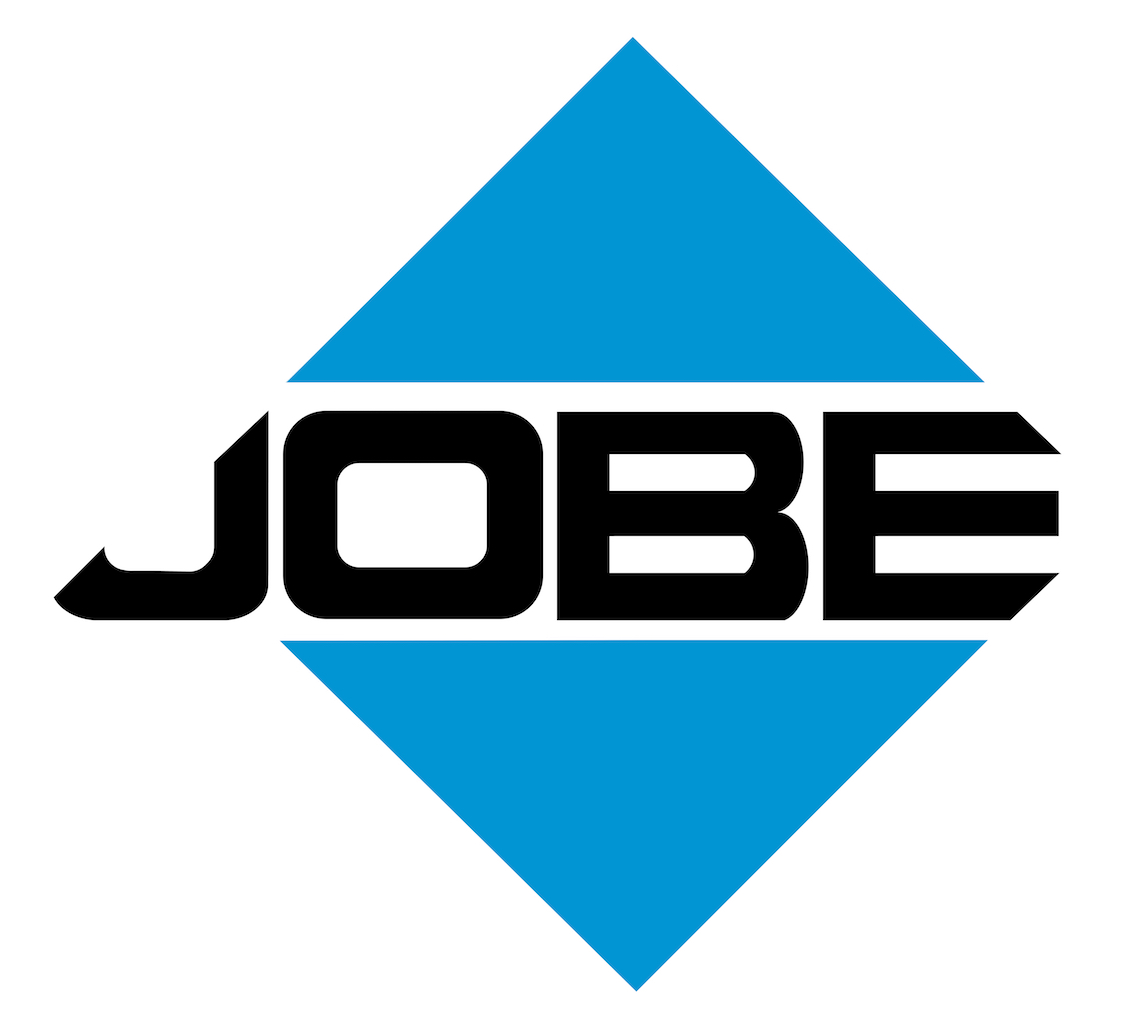Products Services Projects Careers

# Calculators

## Concrete Calculators

Volume Calculator

Width (Feet):
Length (Feet):
Thickness:
Thickness in:
Inches
Feet

Cubic Yards Required:

Block Wall Calculator

Height (Feet):
Length (Feet):
Block Size:
8 inch
12 inch

Cubic Yards Required:

Column Calculator

Height (Feet):
Diameter
Diameter in:
Inches
Feet

Cubic Yards Required:

## Asphalt & Aggregate Calculators

Asphalt Calculator

Width (Feet):
Length (Feet):
Thickness:
Thickness in:
Inches
Feet
Density:
Density in:
Lbs/Cu Ft
Lbs/Cu Yd

Tons Required:

Aggregate Calculator

Width (Feet):
Length (Feet):
Thickness:
Thickness in:
Inches
Feet
Density:
Density in:
Lbs/Cu Ft
Lbs/Cu Yd

Tons Required:
 Note: There are many components that may have an effect on the precise tonnage and yardage needed for every situation. Please feel free to contact Jobe Materials for a personal consultation regarding your particular circumstance.

## Conversions

### Area

Square Inch = 0.007 sq. ft = 6.45 sq cm
Square Foot = 144 sq in. = 929.03 sq cm
Square Yard = 9 sq. ft = 0.836 sq m
Square Rod = 30.25 sq yds
Acre = 4,840 sq yds = 43,560 sq ft = 160 sq rods = 4.047 sq m = 0.405 hectare
Hectare = 10,000 sq m = 2.47 acres
Square Mile = 640 acres = 2.59 sq k = 1 section
Section = 1 sq mile = 640 acres = 2.59 sq k

### Volume

Cubic inch = 0.00058 cu ft = 16.4 cu cm
Cubic foot = 1,728 cu in = 0.037 cu yd = 0.028 cu m
Cubic Yard = 27 cu ft = 0.765 cu m

### Volume Conversion Factors

Cu Ft x 7.48 = gallons
Cu Ft x 62.4 = lbs. of water
Gallons x 8.333 = lbs of water
Gallons x 0.1337 = cu ft
Cu In / 1,728 = cu ft
Cu Yd x 27 = cu ft
Cu Ft / 27 = cu yds

### Length

Inch = 1/12 or 0.083 foot = 2.54 cm = 25.4 mm
Foot = 12 in. = 0.3048 m = 30.48 cm
Yard = 36 in. = 3 ft = 0.9144 m
Rod = 16.5 feet = 5.5 yds = 5.03 m
Furlong = 22 yds
Mile = 1,760 yds = 5,280 ft = 1.61 km = 8 furlongs = 80 chains

### Weight

Gram = 15.43 grains = 1,000 mg
Ounce = 28.35 grams = 437.5 grains
Pound = 16 oz = 7,000 grains = 454 g
Kilogram = 1,000 grams = 2.205 lbs
Ton (short) = 2,000 lbs = 0.907 metric tons
Ton (long) = 2,240 lbs = 1.016 metric tons

### Metric Prefix Definitions

Mega = 1,000,000
Micro = 0.000001
Kilo = 1,000
Hecto = 100
Deca = 10
Milli = 0.001
Centi = 0.01
Deci = 0.1
Basic Metric Unit = 1